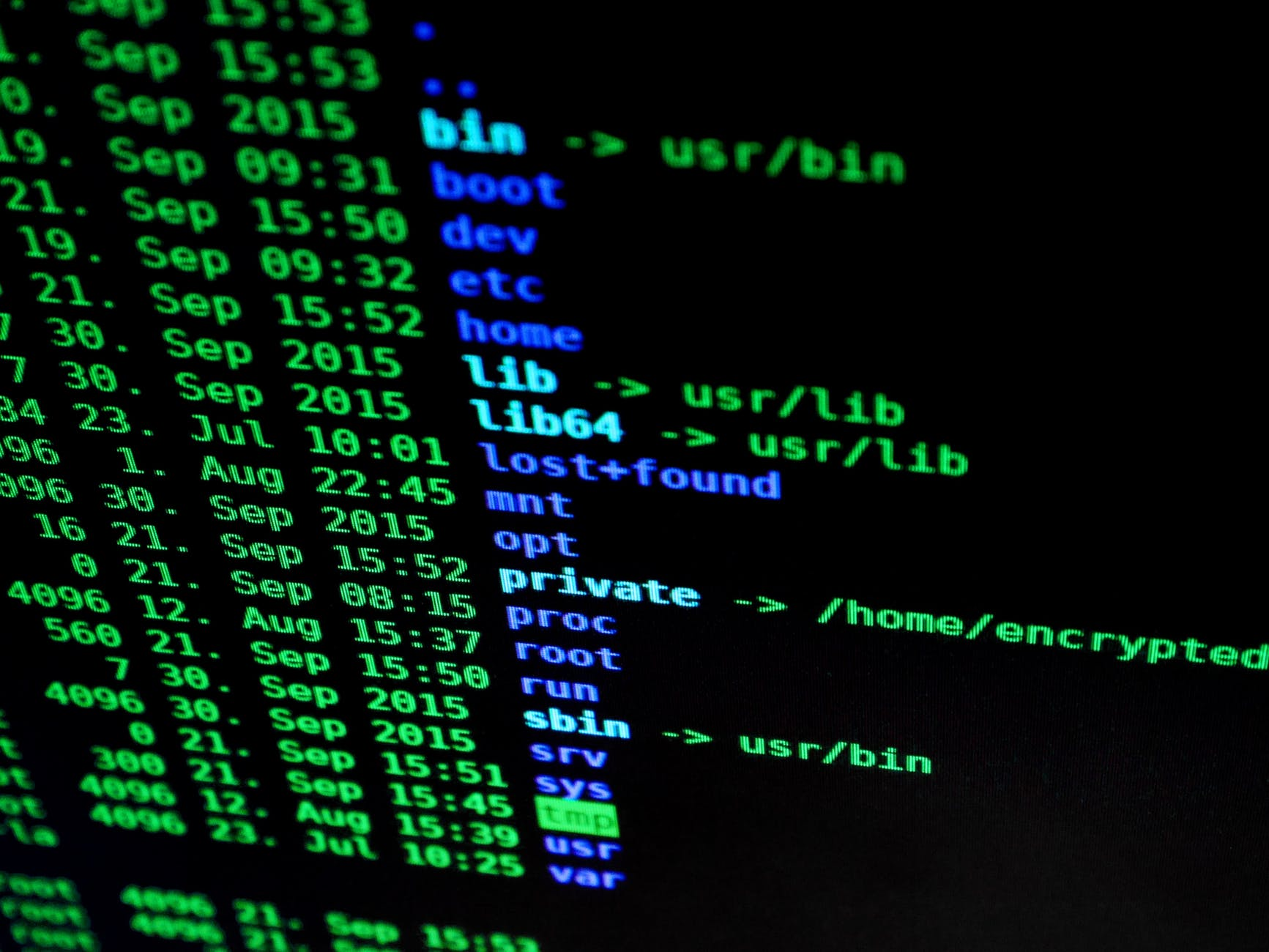# Logical Flow in Python

Applications often have to make decisions and to do this they need a set of conditions to him them decide what to do. Python, like all programming languages, has tools that allow the application to execute various actions based on conditions. In this post, we will look at the use of if statements.

If Statement DefineAn if statement is a statement used in Python that determines when an action should happen. At a minimum, an if statement should have two parts to it. The first part is the condition and the second part is the action that Python performs if the condition is true. Below is what this looks like

```if SOMETHING IS TRUE:
DO THIS```

This is not the most beautiful code but this is the minimum for a if statement in Python.

If Statement

Below is the actual application of the use of an if statement

``` number=5
if number ==5:
print("Correct")

Correct```

In the code above we created a variable called number and set its value to 5. Then we created the if statement by setting the condition as “if number equals 5” the action for this being true was prin the string “correct”, which Python does at the bottom.

Notice the double equal sign used in the if statement. The double equal sign is used for relational equality while the single equal sign is used for assigning values to variables. Also, notice the colon at the end of the if statement. This must be there in order to complete the code.

There is no limit to the number of tasks an if statement can perform. In to code above the, if statement only printed “Correct” however, we can put many more print functions or other actions as shown below.

```number=5
if number ==5:
print("Correct")
print("Done")

Correct
Done```

All that is new above is that we have two print statements that were executed because the if statement was true.

Multiple Comparisons

In the code above python only had to worry about whether number equaled 5. However, we can have Python make multiple comparisons as well below is an example.

```number=5
if (number>0) and (number<10):
print("Correct")
print("Finished")

Correct
Finished```

Now for the print functions to execute the number has to be greater than 0 but less than 10. If you change the number to something less than 0 or greater than 10 nothing will happen when you run the code because the conditions were not met. In addition, if you type in a letter like ‘a’ you will get an error message because Python is anticipating a number and not a string.

If Else Statements

The use of an else clause in an if statement allows for an alternative task to be executed if the first conditions in the if statement are not met. This allows the application to do something when the conditions are not met rather than leaving a blank screen. Below we modify our code in the previous example slightly so that something happens because of the else clause in the if statement.

```number=15
if (number>0) and (number<10):
print("Correct")
print("Finished")
else:
print("Number is out of range")

Number is out of range```

In this code above we did the following

1. we set our number to 15
2. We created an if statement that searches for a number greater than 0 and less than 10.
3. If the conditions in step 2 are true we print the two statements
4. If the conditions in step 2 are not met we print the statement after the else clause
5. Our number was 15 so Python printed the statement after the else clause

It can get much more complicated and powerful than this but I think this is clear and enough for now.

Conclusion

This post provided an introduction to the if statement in Python. THe if statement will execute a command based on one or more conditions. You must be careful with the syntax. Lastly, you can also include an alternative task with the else clause if the conditions are not met.